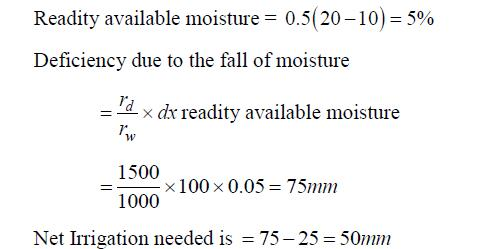Courses

# Test: Hydrology & Irrigation- 2

## 20 Questions MCQ Test GATE Civil Engineering (CE) 2022 Mock Test Series | Test: Hydrology & Irrigation- 2

Description
This mock test of Test: Hydrology & Irrigation- 2 for GATE helps you for every GATE entrance exam. This contains 20 Multiple Choice Questions for GATE Test: Hydrology & Irrigation- 2 (mcq) to study with solutions a complete question bank. The solved questions answers in this Test: Hydrology & Irrigation- 2 quiz give you a good mix of easy questions and tough questions. GATE students definitely take this Test: Hydrology & Irrigation- 2 exercise for a better result in the exam. You can find other Test: Hydrology & Irrigation- 2 extra questions, long questions & short questions for GATE on EduRev as well by searching above.
QUESTION: 1

Solution:
QUESTION: 2

Solution:
QUESTION: 3

### In a linear reservoir, the

Solution:
QUESTION: 4

Mukingum’s method for the routing of flood

Solution:
QUESTION: 5

A lysimeter is used to measure

Solution:
QUESTION: 6

Lysimeter and Tensiometer are used to measure, respectively, one of the following groups of quantities:

Solution:
QUESTION: 7

If the discharge required for different crops is 0.4 cumecs in the field and the capacity factor and time factors are 0.8 and 0.5, respectively, then what is the design discharge of the distributary at its head?

Solution: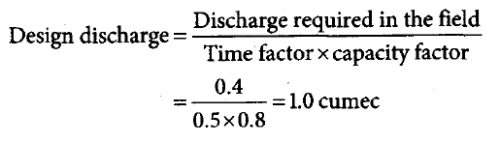QUESTION: 8

In a canal irrigation project, 76% of the culturbale command area (CCA) remained without water during Kharif season; and 58% of CCA remained without water during Rabin season in a particular year. Rest of the areas got irrigated in each crop, respectively. What is the intensity of irrigation for the project in the year?

Solution: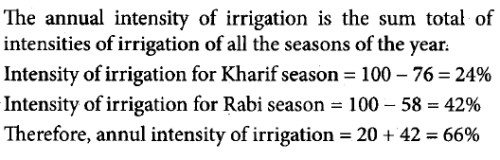QUESTION: 9

The wave height, in meters, generated on the surface of a reservoir, having a fetch length F = 30 km, due to wind blowing on the surface of the reservoir at a velocity of 30 km/h is

Solution: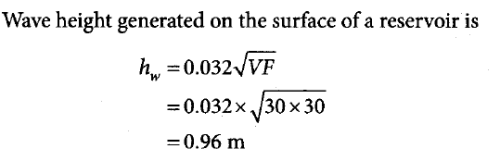QUESTION: 10

An identified source of irrigation water has ion concentrations of Na+, Ca++, and Mg++ as 20, 10, and 8 mEq/L (milliequivalent per liter), respectively. The sodium absorption ratio (SAR) of this water is approximately

Solution: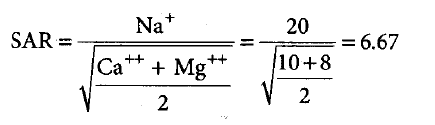QUESTION: 11

A canal was designed to supply irrigation needs of 1200 ha of land growing rice of 140 days base period having a delta of 134 cm. If this canal water is used to irrigate wheat of base period 120 days having delta of 52 cm, the area (in ha) that can be irrigated is

Solution: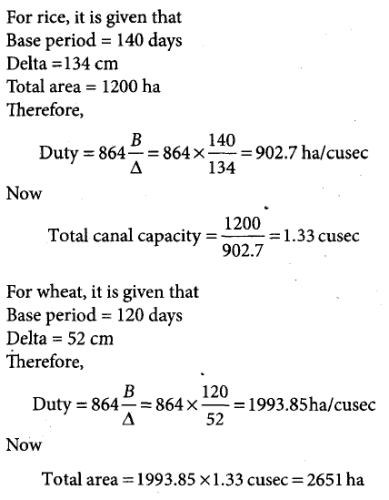QUESTION: 12

What is the moisture depth available for evapotranspiration in root zone of 1 m depth soil, if dry weight of soil is 1.5 g/cm3, field capacity is 30%, and permanent wilting point is 10%? A. 450 mm B. 300 mm C. 200 mm D. 150 mm

Solution: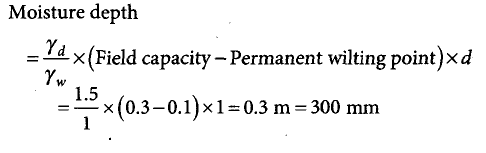*Answer can only contain numeric values
QUESTION: 13

During a particular stage of the growth of a crop, consumptive use of water is 2.8 mm/day. If the amount of water available in the soil is 25% of 80 mm depth of water, the frequency of irrigation is ________ days.

Solution: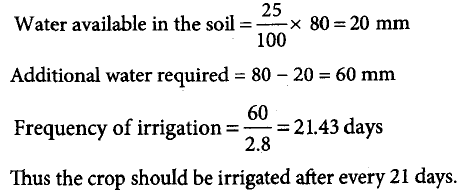*Answer can only contain numeric values
QUESTION: 14

The maximum height of a low gravity dam of elementary profile made of concrete of relative density 2.5 and safe allowable stress of foundation material 3.87 MPa without considering the uplift force is about _________ m.

Solution: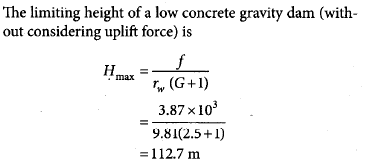QUESTION: 15

A 252 km2 catchment area has a 6-h unit hydrograph which is a triangle with time base of 35 h. What is the peak discharge of the DRH due to 5 cm effective rainfall in 6 h from that catchment?

Solution:
QUESTION: 16

The time of concentration at the outlet in an urban catchment area of 1.5 km2 with a runoff coefficient of 0.42 is 28 min. The maximum depth of rainfall with a 50 year return period for this time of concentration is 48 mm. What is the peak flow rate at the outlet for this return period?

Solution:
*Answer can only contain numeric values
QUESTION: 17

A unit hydrograph for a watershed is triangular in shape with base period of 20 h. The area of the watershed is 500 ha. The peak discharge in m3/h is __________.

Solution:
QUESTION: 18

A channel of bed slope 0.0009 carries a discharge of 30 m3/s when the depth of flow is 1.0 m. What is the distance carried by an exactly similar channel at the same depth of flow if the slope is decreased to 0.0001?

Solution:
QUESTION: 19

A field was supplied water from an irrigation tank at a rate of 120 L/s to irrigate an area of 2.5 ha. The duration of irrigation is 8 h. It was found that the actual delivery at the field, which is about 4 km from the tank, was 100 L/s. The runoff loss in the field was estimated as 800 m3. Then the application efficiency will be

Solution:
QUESTION: 20

The following data were recorded from an irrigated field:

1. Field capacity: 20%

2. Permanent wilting point: 10%

3. Permissible depletion of available soil moisture: 50%

4. Dry unit weight of soil: 1500 kgf/m3

5. Effective rainfall: 25 mm

Based on these data, the net irrigation required per meter depth of soil will b

Solution: